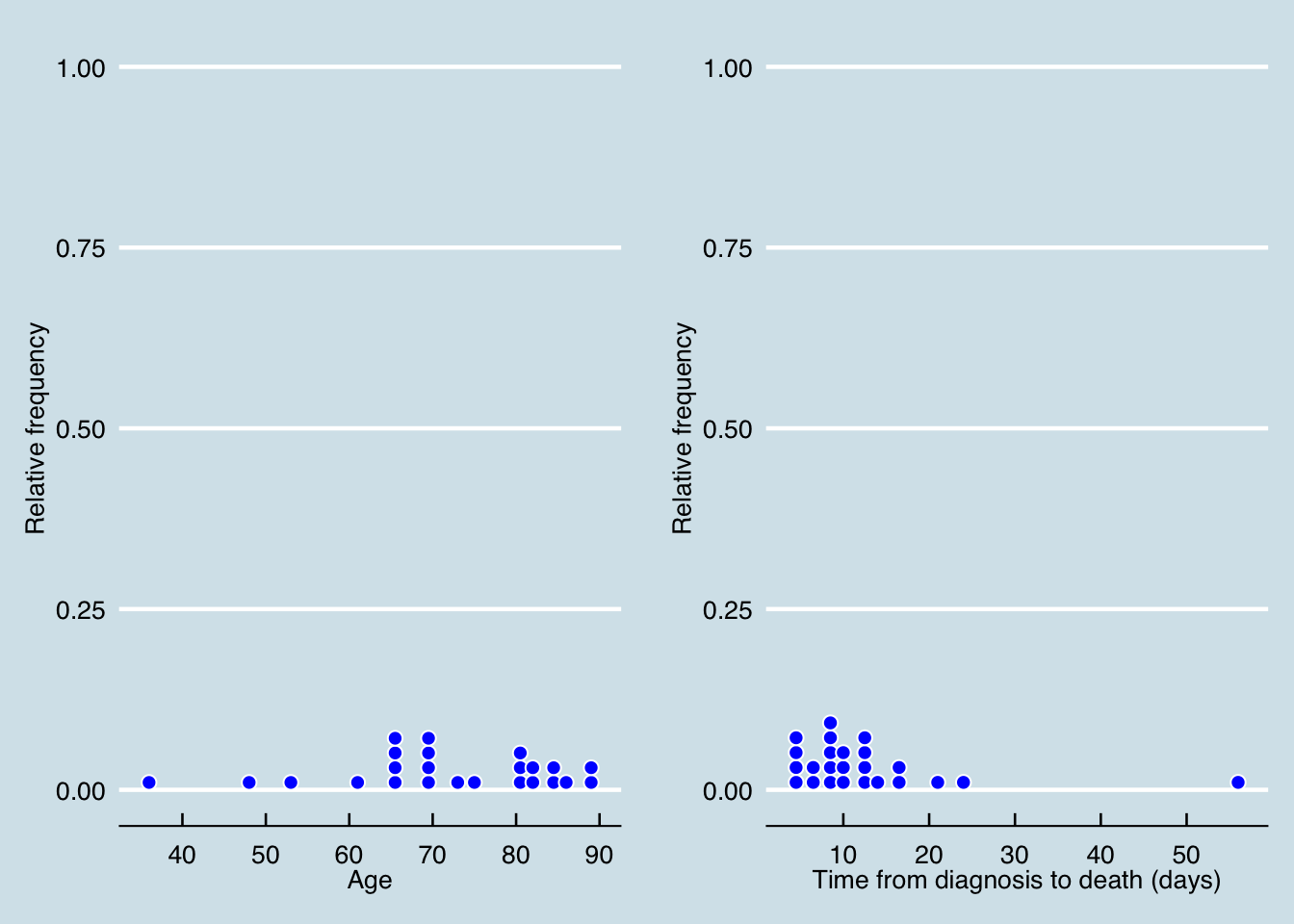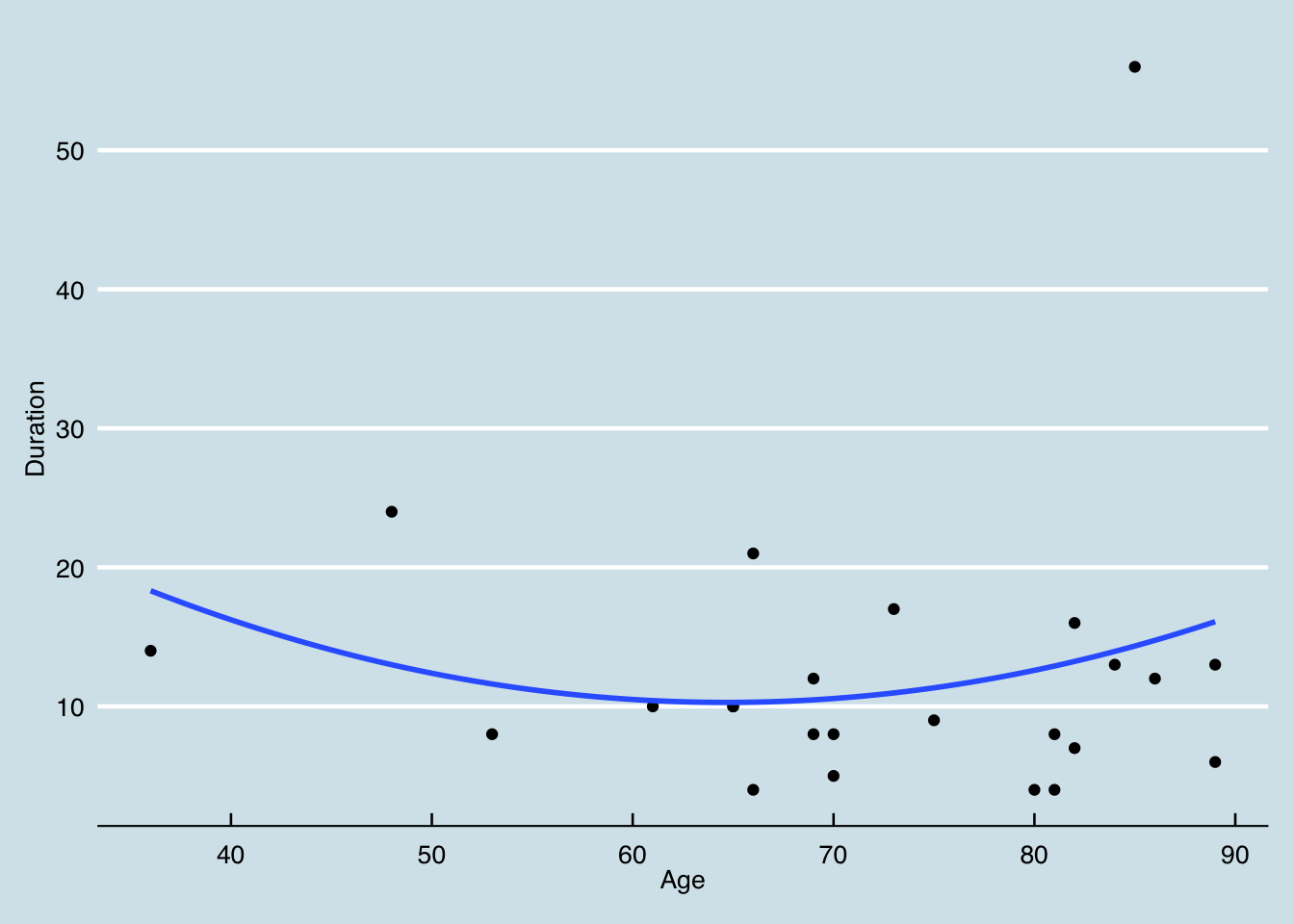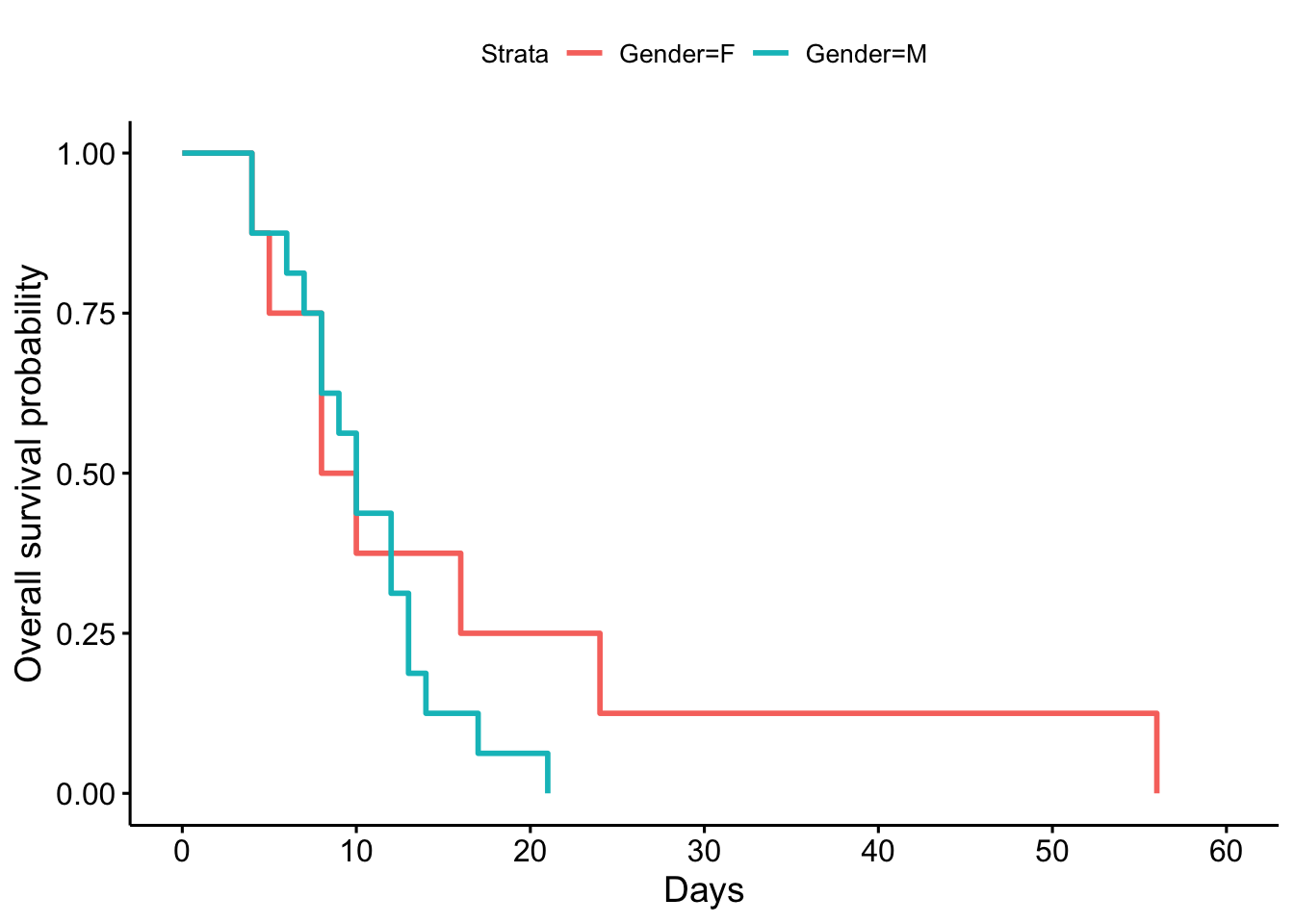### Source: https://www.bloomberg.com/news/articles/2020-01-23/several-china-coronavirus-victims-didn-t-even-have-a-fever

library(ggplot2); library(gridExtra); library(ggthemes); library(table1)
##
## Attaching package: 'table1'
## The following objects are masked from 'package:base':
##
##     units, units<-
# Updated data published by Bloomberg
t = "/Users/tuannguyen/Dropbox/Temp Files/Coronavirus/Coronavirus data II.csv"
cvr\$Status = 1
table1(~Age + Gender + Duration, data=cvr)
Overall
(n=24)
Age
Mean (SD) 71.9 (13.3)
Median [Min, Max] 71.5 [36.0, 89.0]
Gender
F 8 (33.3%)
M 16 (66.7%)
Duration
Mean (SD) 12.5 (10.6)
Median [Min, Max] 10.0 [4.00, 56.0]

# Visualizing data

# Distribution of age at death
p1 = ggplot(data=cvr, aes(x=Age)) + geom_dotplot(col="white", fill="blue") + labs(x="Age", y="Relative frequency") + theme_economist()

p2 = ggplot(data=cvr, aes(x=Duration)) + geom_dotplot(col="white", fill="blue") + labs(x="Time from diagnosis to death (days)", y="Relative frequency") + theme_economist()

grid.arrange(p1, p2, ncol=2)
## stat_bindot() using bins = 30. Pick better value with binwidth.
## stat_bindot() using bins = 30. Pick better value with binwidth.# Age and duration

ggplot(data=cvr, aes(x=Age, y=Duration), col="blue") + geom_point() + geom_smooth(se=F, method="lm", formula=y~x+I(x^2)) + theme_economist()# Survival analysis

library(survival); library(survminer)
## Loading required package: ggpubr
## Loading required package: magrittr
s = survfit(Surv(Duration, Status) ~ Gender, data = cvr)
ggsurvplot(s, xlab = "Days", ylab = "Overall survival probability")